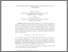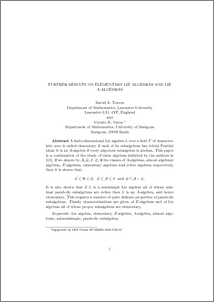# Further results on elementary Lie algebras and Lie A-algebras.

Towers, David A. and Varea, Vicente R. (2013) Further results on elementary Lie algebras and Lie A-algebras. Communications in Algebra, 41 (4). pp. 1432-1441. ISSN 0092-7872Preview
PDF (Further_results_on_elementary_Lie_algebras_and_Lie_A-algebras.pdf)
Further_results_on_elementary_Lie_algebras_and_Lie_A-algebras.pdf

## Abstract

A finite-dimensional Lie algebra L over a field F of characteristic zero is called elementary if each of its subalgebras has trivial Frattini ideal; it is an A-algebra if every nilpotent subalgebra is abelian. This paper is a continuation of the study of these algebras initiated by the authors in a previous paper. If we denote by $\mathcal{A}$, $\mathcal{G}$, $\mathcal{E}$, $\mathcal{L}$, $\Phi$ the classes of A-algebras, almost algebraic algebras, E-algebras, elementary algebras and $\phi$-free algebras respectively, then it is shown that: \mathcal{L} \subset \Phi \subset \mathcal{G} \mathcal{L} \subset \mathcal{A} \subset \mathcal{E} \mathcal{G} \cap \mathcal{A} = \mathcal{L}. It is also shown that if L is a semisimple Lie algebra all of whose minimal parabolic subalgebras are $\phi$-free then L is an A-algebra, and hence elementary. This requires a number of quite delicate properties of parabolic subalgebras. Finally characterisations are given of $E$-algebras and of Lie algebras all of whose proper subalgebras are elementary.

Item Type:
Journal Article
Journal or Publication Title:
Communications in Algebra
The final, definitive version of this article has been published in the Journal, Communications in Algebra, 41 (4), 2013, © Informa Plc
Uncontrolled Keywords:
/dk/atira/pure/researchoutput/libraryofcongress/qa
Subjects:
Departments:
ID Code:
27159
Deposited By:
Deposited On:
05 Oct 2009 14:09
Refereed?:
Yes
Published?:
Published